## B.5 Multi-Antenna channel models

36.1413GPPBase Station (BS) conformance testingEvolved Universal Terrestrial Radio Access (E-UTRA)Release 17TS

The MIMO channel correlation matrices defined in B.5 apply for the antenna configuration using uniform linear arrays at both UE and eNodeB.

## B.5.1 Definition of MIMO Correlation Matrices

Table B.5.1-1 defines the correlation matrix for the eNodeB:

Table B.5.1-1: eNodeB correlation matrix

 One antenna Two antennas Four antennas eNode B Correlation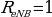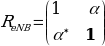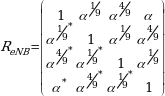Table B.5.1-2 defines the correlation matrix for the UE:

Table B.5.1-2: UE correlation matrix

 One antenna Two antennas Four antennas UE Correlation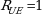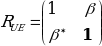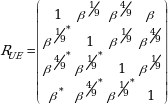Table B.5.1-3 defines the channel spatial correlation matrix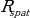. The parameters α and β in Table B.5.1-3 defines the spatial correlation between the antennas at the eNodeB and UE.

Table B.5.1-3:correlation matrices

 1×2 case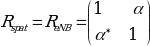2×2 case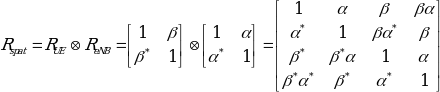2×4 case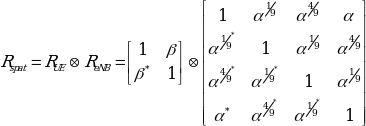4×4 case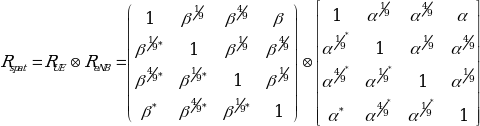For cases with more antennas at either eNodeB or UE or both, the channel spatial correlation matrix can still be expressed as the Kronecker product of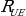and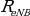according to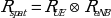.

## B.5.2 MIMO Correlation Matrices at High, Medium and Low Level

The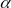and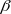for different correlation types are given in Table B.5.2-1.

Table B.5.2-1: Correlation for High Medium and Low Level

 Low correlation Medium Correlation High Correlation 0 0 0.9 0.3 0.9 0.9

The correlation matrices for high, medium and low correlation are defined in Table B.5.2-2, B.5.2-3 and B.5.2-4 as below.

The values in Table B.5.2-2 have been adjusted for the 2×4 and 4×4 high correlation cases to insure the correlation matrix is positive semi-definite after round-off to 4 digit precision. This is done using the equation: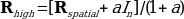Where the value “a” is a scaling factor such that the smallest value is used to obtain a positive semi-definite result. For the 2×4 high correlation case, a=0.00010. For the 4×4 high correlation case, a=0.00012.

The same method is used to adjust the 4×4 medium correlation matrix in Table B.5.2-3 to insure the correlation matrix is positive semi-definite after round-off to 4 digit precision with a =0.00012.

Table B.5.2-2: MIMO correlation matrices for high correlation

 1×2 case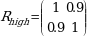2×2 case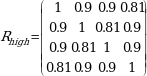2×4 case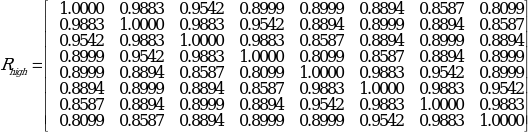4×4 case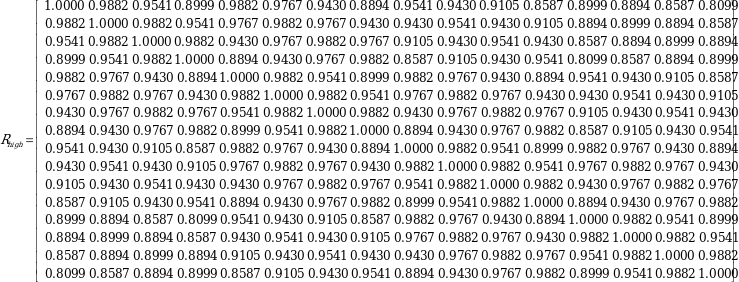Table B.5.2-3: MIMO correlation matrices for medium correlation

 1×2 case N/A 2×2 case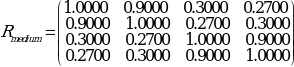2×4 case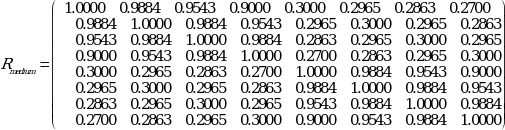4×4 case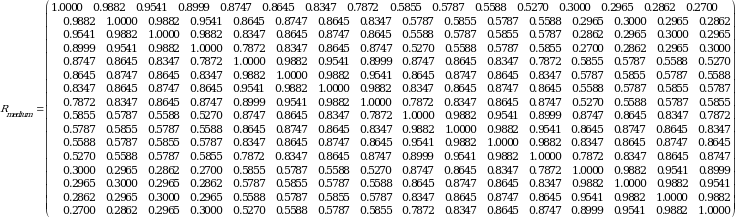Table B.5.2-4: MIMO correlation matrices for low correlation

 1×2 case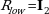1×4 case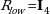2×2 case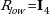2×4 case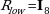4×4 case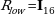In Table B.5.2-4,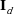is a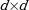identity matrix.

NOTE: For completeness, the 1×2 cases were defined for high, medium and low correlation but for Rel-8 onwards for 1Tx, performance requirements exist only for low correlation.